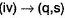Courses

# Test: Diols And Polyols

## 19 Questions MCQ Test Chemistry Class 12 | Test: Diols And Polyols

Description
This mock test of Test: Diols And Polyols for Class 12 helps you for every Class 12 entrance exam. This contains 19 Multiple Choice Questions for Class 12 Test: Diols And Polyols (mcq) to study with solutions a complete question bank. The solved questions answers in this Test: Diols And Polyols quiz give you a good mix of easy questions and tough questions. Class 12 students definitely take this Test: Diols And Polyols exercise for a better result in the exam. You can find other Test: Diols And Polyols extra questions, long questions & short questions for Class 12 on EduRev as well by searching above.
QUESTION: 1

### Only One Option Correct Type Direction (Q. Nos. 1-8) This section contains 8 multiple choice questions. Each question has four choices (a), (b), (c) and (d), out of which ONLY ONE is correct. Consider the following reaction, CH2 = CH2 + Cl2 - H2O → ClCH2 — CH2OH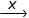HOCH2CH2OH Q.  The most appropriate reagent X for the above reaction is

Solution:

A slightly weaker base (NaHCO3) is used in the second step SN2 reaction otherwise oxirane formation would be preferred.

QUESTION: 2

### Which is the most suitable condition for the following transformation?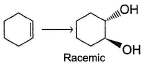Solution:

Epoxide, upon hydrolysis in either acidic or alkaline medium gives anti, vicinal diol.

QUESTION: 3

### What is the increasing order of solubility of the following in water?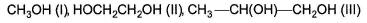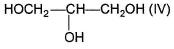Solution:

Greater the number of hydroxy groups, greater the solubility in water. Between (II) and (III), (III) is slightly less soluble due to greater hydrophobic alkyl fraction.

QUESTION: 4

How many different diols product are formed in the following reaction?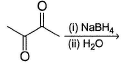Solution:

At planar carbonyl carbons, hydride ion attacks from both sides giving both stereomers. Hence, the product diols would be all stereomers of 2, 3 - butanediol.

QUESTION: 5

Which does not form dihalide when treated with ethylene glycol?

Solution:

(d) PI3 does not form di iodide with vicinal diols.

QUESTION: 6

What is the major organic product of the following reaction?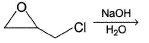Solution: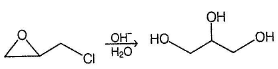Both oxirane hydrolysis and halide substitution (SN2) take place.

QUESTION: 7

What is formed as the major product in the reaction given below?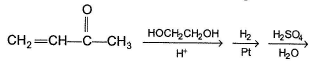Solution: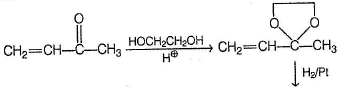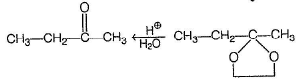QUESTION: 8

The major organic product formed below is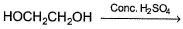Solution:

Ethylene glycol on heating with concentrated H2SO4 gives 1 , 4-dioxane

*Multiple options can be correct
QUESTION: 9

One or More than One Options Correct Type

Direction (Q. Nos. 9-14) This section contains 6 multiple choice questions. Each question has four choices (a), (b), (c) and (d), out of which ONE or MORE THAN ONE are correct.

Q.

Which of the following reaction(s) gives diols?

Solution:

(a) Alkaline KMnO4 gives syn, vicinal diol with alkene.
(b) Here, oxirane is formed as the major product.
(c) Epoxidation followed by hydrolysis gives diol.
(d) Dihalide formed in the first step undergoes SN2 reaction with NaHCO3 giving diol.

*Multiple options can be correct
QUESTION: 10

Which reaction(s) gives dioxane?

Solution:

(a) Vicinal diols, on heating with concentrated H2SO4 gives dioxane as the major product.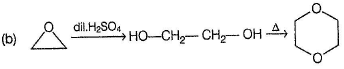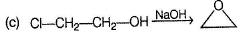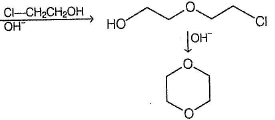(d) Diols do not give dioxane in alkaline medium.

*Multiple options can be correct
QUESTION: 11

Which reaction(s) given below gives dicarbonyl?

Solution:

Syn vicinal diols undergo oxidative cle avage with HIO4 giving diols, Option (b) and option (c) are anti diol and have restricted rotation.

*Multiple options can be correct
QUESTION: 12

In the following rearrangement, possible product(s) is/are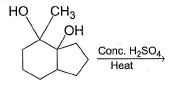Solution:

It is a Pinacol-pinacolone rearrangement.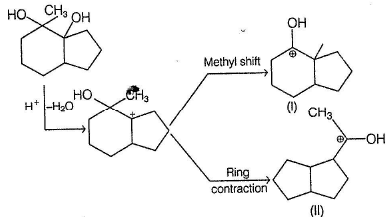(I) on deprotonation gives (a) while (II) on deprotonation gives (b).

*Multiple options can be correct
QUESTION: 13

Which reaction(s) given below gives glycerol?

Solution: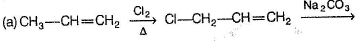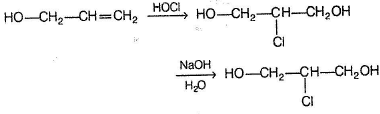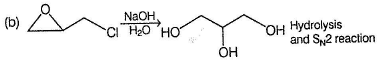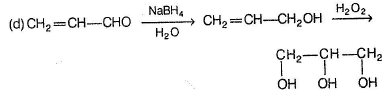*Multiple options can be correct
QUESTION: 14

In the following rearrangement, reaction possible product(s) is/are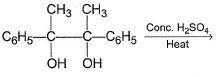Solution:

Pinacol-pinacolone rearrangemen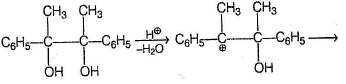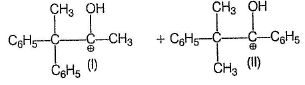Deprotonation of (I) gives (a) while deprotonation of (II) gives (c).

QUESTION: 15

Comprehension Type

Direction (Q. Nos. 15-17) This section contains a paragraph, describing theory, experiments, data, etc. Three questions related to the paragraph have been given. Each question has only one correct answer among the four given options (a), (b), (c) and (d).

Passage

Glycol when heated with concentrated H2SO4, undergo a variety of reactions as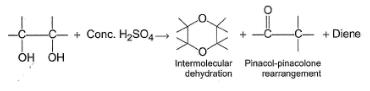Nature of predominant product depends upon the nature of other groups present at the two α-carbons.

Q.

Which is most likely to undergo intermolecular dehydration to give dioxane or substituted dioxane?

Solution:

Vicinal diols which is not tertiary, prefer to form dioxane while tertiary diols prefer to undergo Pinacol-pinacolone rearrangement.

The diol is not tertiary, hence more likely to form dioxane.

QUESTION: 16

Glycol when heated with concentrated H2SO4, undergo a variety of reactions asNature of predominant product depends upon the nature of other groups present at the two α-carbons.

Q.

Which of the following is most likely to undergo Pinacol-pinacolone rearrangement?

Solution:

Vicinal diols which is not tertiary, prefer to form dioxane while tertiary diols prefer to undergo Pinacol-pinacolone rearrangement.
It is tertiary diol, undergo Pinacol-pinacolone rearrangeme.

QUESTION: 17

Glycol when heated with concentrated H2SO4, undergo a variety of reactions asNature of predominant product depends upon the nature of other groups present at the two α-carbons.

Q.

Which diol given below is most likely to form diene in the above reaction?

Solution:

Vicinal diols which is not tertiary, prefer to form dioxane while tertiary diols prefer to undergo Pinacol-pinacolone rearrangement.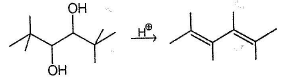QUESTION: 18

Matching List Type

Direction (Q . Nos. 18 and 19) C hoices for the correct combination of elements from Column I and Column II are given as options (a), (b), (c) and (d), out of which one is correct.

Q.

Match the reactant from Column I with the reaction(s) from Column II and mark the correct option from the codes given below.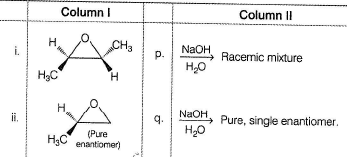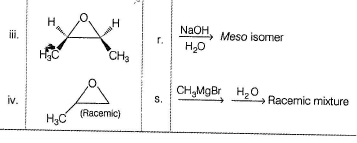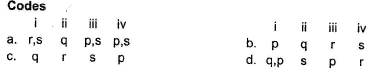Solution: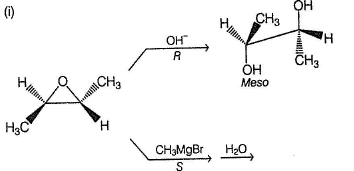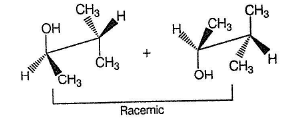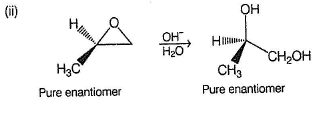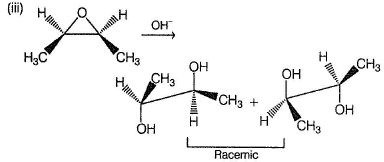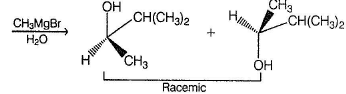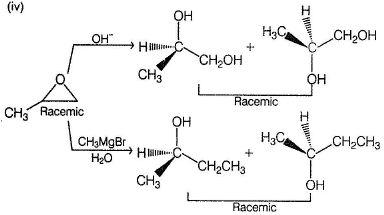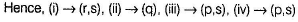QUESTION: 19

Match the compounds in Column I with their reaction and product(s) in Column II and mark the correct option from the codes given below.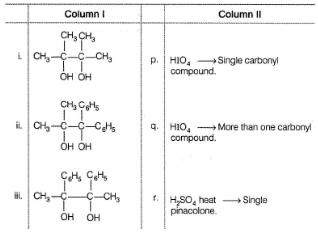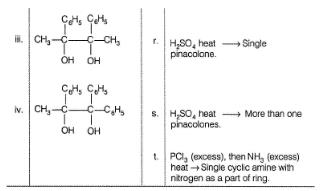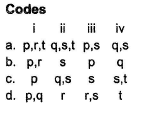Solution:

(i) It is a symmetrical, 3° diol, gives (CH3)2CO with HIO4, a single pinacolone (CH3)3C —COCH3 and a single cyclic a mine :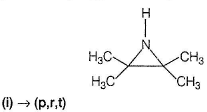(ii) With HIO4 gives (CH3)2CO and (C6H5)2CO. (ii) is unsymmetrical, 3° diol, gives more than one pinacolone in rearrangement reaction. However it gives a single cyclic amine.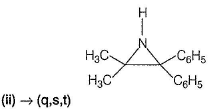(iii) with HIO4 gives a single ketone-acetophenone. It gives two different pinacolone in rearrangement reaction.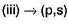(iv) It is unsymmetrical, 3° diol, gives two different ketones with HIO4 and more than one pinacolone in rearrangement reaction.